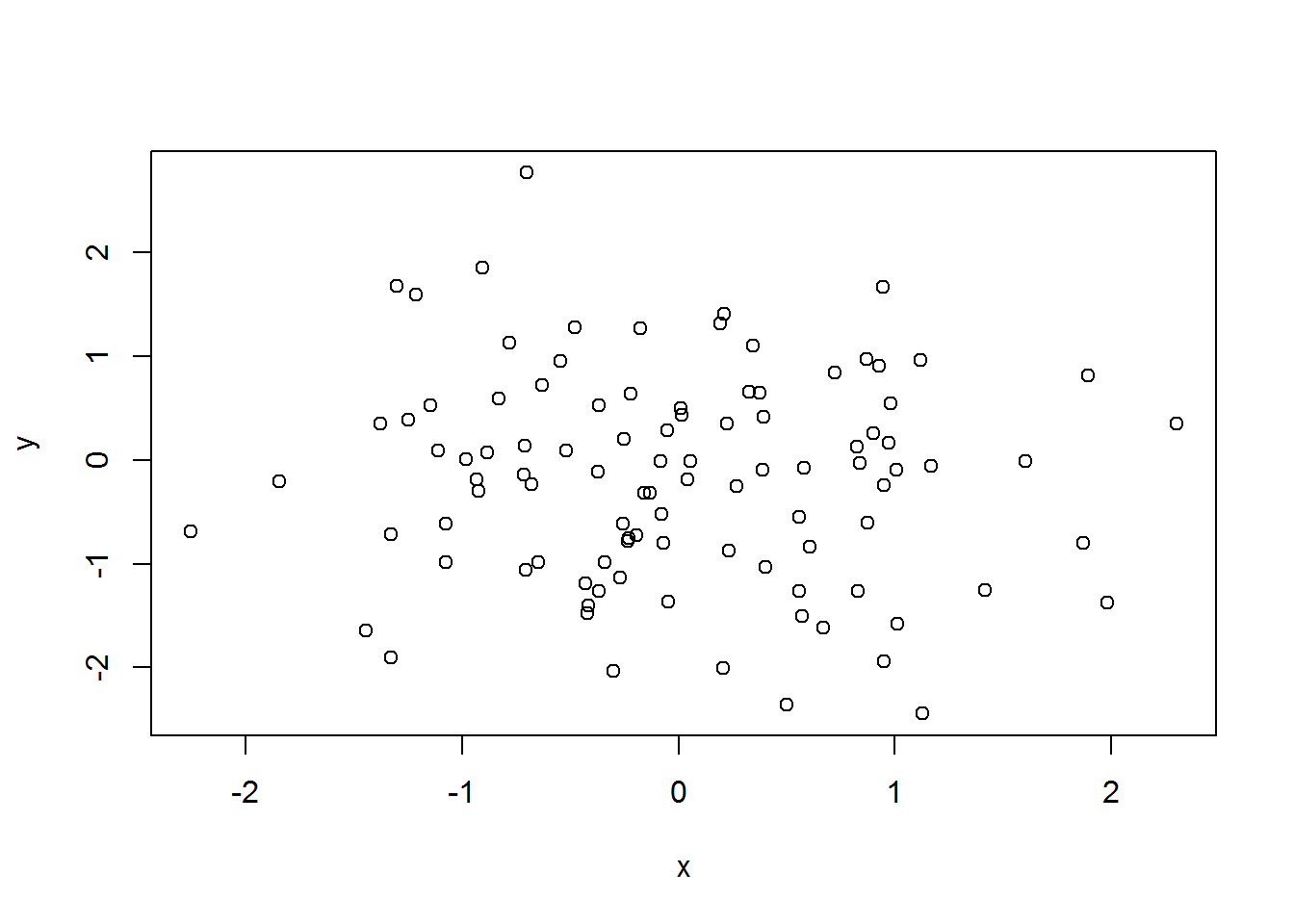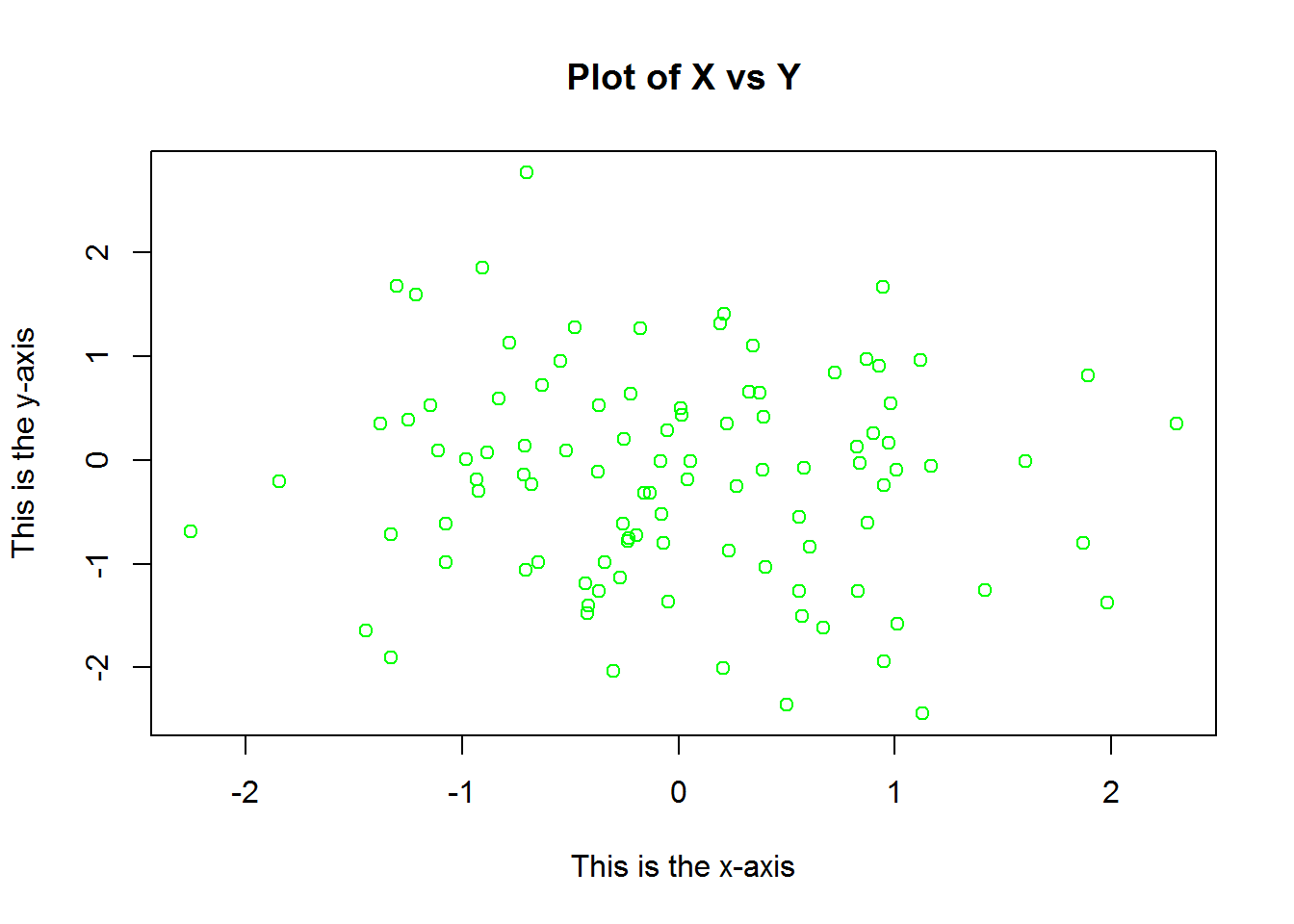This week is a continuation of our introduction to R. You will review some key function concepts, alongside being introduced to basic calculations, simulation, and graphing.

# 1. Introduction to R programming (Week 2)

## 1.1a Review , c() and vectors.

Let’s review the concatenate function. Remember what it does?

x <- c(1,6,2)

x
##  1 6 2
y <- c(1,4,3)

y 
##  1 4 3

We use c() here to create two numeric vectors. I assume that you remember the logic behind vectors, but remember that they hold different types of data. Say we have a vector that is large, or unknown to us. R allows us to describe vector attributes as well as manipulate them in higher order operations. Try typing the code below:

length(x)
##  3
length(y)
##  3

The length() function tells us the long-wise dimension of our vector! Let’s try something new with vectors…try typing the code below.

x + y
##   2 10  5
z = x + y

d = x / y

s = x - y

m = x * y

Go ahead and print (implicitly or explicitly) your new vectors! Do you understand what each line of code did?

## 1.1b Workspace cleanup using code.

While one can utilize R Studio to make clean up easier, lets try cleaning up our work space through the R console. Type the code below.

ls()
##  "d" "m" "s" "x" "y" "z"

The list objects “ls()” function displays all objects that are currently in working memory. As I showed you before, it is easy to get rid of these with the environment tab in R Studio, but how can we do it without using that method? Lets try removing just the x and y vector objects

rm(x, y)

ls()
##  "d" "m" "s" "z"

The remove objects “rm()” function simply removed x and y in this case. We can see that they are missing in both the R Studio environment window and by using the ls() function. What if we want to quickly remove all objects in memory?

rm(list=ls())

ls()
## character(0)

Woah! We can see that everything is gone. Do you understand the logic of the code that was used? If not, that is okay. Remember that when you typed out ls(), it printed out what appeared to be a character vector of each object in working memory. Like any operation we have performed before, there is no reason why we couldn’t place this output into its own vector object! The code list = ls() does this nicely. The object called list is told to house the names of all objects in current working memory. If we apply the rm() function to this newly created object, it will read all current objects in working memory and remove them, including your newly created list vector.

## 2 matrix(), or how I learned to love matrix algebra…

Remember before that I mentioned the idea of vectors as objects. Additionally, it was communicated that vectors are not the only type of object that R recognizes, but that vectors were essentially the simplest type of object one can work with. A slightly more complex object is the matrix object. What do you think separates a matrix object from a vector? Lets try creating one.

x = matrix(data = c(1,2,3,4), nrow=2, ncol=2)

x
##      [,1] [,2]
## [1,]    1    3
## [2,]    2    4

The result is probably what you suspected all along. Matrices are simply two vectors that are combined into columns and rows (think of spreadsheets!). There are a few new arguments introduced to us when creating the matrix “x”. The data argument tells the matrix() function what numeric data to use, while the nrow argument indicates the number of rows and the ncol argument indicates the number of columns. Matrices in R utilize standard matrix algebra rules, so be mindful when combining different types of vectors.

Like vectors, one can use mathematical operations to manipulate the values inside matrices. Lets introduce you to some additional mathematical operations in R.

sqrt(x)
##          [,1]     [,2]
## [1,] 1.000000 1.732051
## [2,] 1.414214 2.000000
x^2
##      [,1] [,2]
## [1,]    1    9
## [2,]    4   16

The first line of code uses the sqrt() function to apply the square root to each matrix value. Many simple mathematical operations, or transformations, have built in R functions. The second line of code applies the square exponent to the matrix values. As you can see this is more in line with the addition/subtraction code used above. We can use the ^ operator to exponate a number to any power value.

x^3
##      [,1] [,2]
## [1,]    1   27
## [2,]    8   64
x^5
##      [,1] [,2]
## [1,]    1  243
## [2,]   32 1024
x^10
##      [,1]    [,2]
## [1,]    1   59049
## [2,] 1024 1048576
x^100
##              [,1]         [,2]
## [1,] 1.000000e+00 5.153775e+47
## [2,] 1.267651e+30 1.606938e+60

## 3. Introduction to simulation.

This next series of code is a bit more complicated, yet it demonstrates the power of R. Type the code out below.

x = rnorm(50)

x
##    1.45384171 -0.19103841  1.39956716 -2.12998017 -0.12185415
##    0.35867788  0.15086251  2.05520869  0.60693572 -0.09148398
##   1.17580446 -0.13393359 -0.59751935  1.11272686  0.32823646
##  -1.03776984 -0.43616250  1.88879194  0.32479444  0.48258770
##  -1.24848853 -1.03189990  1.38096551  0.81823893  0.93733366
##  -0.45707274  1.51712713  1.46147254 -0.89019982  3.04979599
##   1.34344364  0.35386833 -0.71181650  0.07721426  1.11496465
##  -0.29515987 -0.19586068 -1.30053305  1.36015662 -0.47281892
##  -0.62030875  1.19331628 -0.62980461  0.93282037  0.03060069
##   0.71369358  1.23072306  1.04007954  1.51377436 -1.15276476

Judging by the printed output it is clear that we have created a numeric vector of length 50, but how did we do this? The rnorm() function is a tool that randomly samples vector values from a normal distribution. The 50 value in the function parentheses indicates that we wanted fifty random samples in our new vector. Remember that the standard normal distribution requires a mean of 0 and a standard deviation of 1, or simply $$N(\mu = 0,\sigma = 1)$$.

When we type the code above, it automatically gives us $$N(0, 1)$$, but it is possible to specify alternative values for $$\mu$$ and $$\sigma$$. Lets try simulating a vector with $$\mu = 50$$ and $$\sigma = .1$$.

y = rnorm(50, mean = 50, sd = .1)

y
##   49.97508 50.14694 49.95523 49.91233 50.07850 49.81777 50.05973
##   50.05932 49.88792 50.15224 49.92573 49.98346 50.05298 50.20256
##  49.98409 50.03889 50.02995 50.20282 50.01180 50.07414 50.05762
##  50.07200 50.06382 49.92074 49.98609 49.93938 50.09910 50.05261
##  50.29566 49.99713 49.84256 50.14406 50.01394 49.92006 50.11388
##  49.95386 50.05413 49.81266 49.99931 50.17520 50.06802 50.02051
##  49.84928 50.05525 49.87623 50.00081 50.02553 50.01613 49.94606
##  49.94190

We have created a new random vector that displays a minimal spread around an average value of 50. Let’s try diving into more familiar statistical analysis for a moment. Let’s try and find the Pearson correlation coefficient for these two randomly simulated vectors.

cor(x, y)
##  0.06120966

I found a correlation coefficient of 0.03847774, which is not very high! Do you notice something odd though? Your calculated correlation coefficient is probably not the same. Go back and run the code to create x and y again. If you pay attention, you will probably notice that your values are bit different each time. Why do you think that is? The rnorm() function, or any simulation/sampling function, works with a pseudo random sampling system.1 What if we want to replicate our own work, or allow others to do so? We can achieve replication for random sampling by using a seed value. The random seed value tells the program to perform the calculations/sampling on a specific random selection value that will produce the same results every time the seed value is used. Let’s create a random seed value.

set.seed(1452)

x = rnorm(50)

mean(x)
##  0.05073796
y = rnorm(50, mean = 50, sd = .1)

mean(y)
##  49.99886
cor(x, y)
##  0.1493827

By specifying the random seed, you should see that you produce the same results as the example above. The set.seed() function can take any numeric value as its random seed value. You should always use a random seed when working with random or simulated quantities in your work. Finally, we used only the rnorm() function to specify simulated vectors from the normal distribution. You are not restricted to only the normal distribution as R has built in function for almost every statistical distribution of interest to a data analyst. Go try and find other types of distribution sample functions.

## 4. Quantities of interest and R functions.

You may of noticed that we utilized a function called mean() in the code above. You probably suspected that this was a simple way of extracting the mean value for a numeric vector, this is correct! Here you will learn how to extract three important quantities of interest, namely mean, variance, and standard deviation.

set.seed(3)

y = rnorm(1000)

mean(y)
##  0.006396535
var(y)
##  0.9961545
sqrt(var(y))
##  0.9980754

Here we quickly created a random vector of 1000 values from a standard normal distribution. The first line of code produces the mean, the second the variance, and the third the standard deviation. Remember that standard deviation ($$\sigma$$) is the square root of variance ($$\sigma^{2}$$), or $$\sqrt{\sigma^{2}}$$. Fortunately, programmers are lazy and have built in a function that performs this calculation for you.

sd(y)
##  0.9980754

This is a good example of the usefulness of functions. Often we get bogged down in writing .do files or R scripts for complex calculations. One can easily write a function that includes these calculations. This saves time and space, while allowing us to share our own methods in a useful and condensed format.

## 4. Introduction to graphing in R

R is well-known for its ability to produce high quality graphics. The downside is that graphing in R has its own considerable learning curve. This section is to quickly introduce you to basic graph production in R. We will go over more complex and interesting graph creation later in the semester.

Lets start by using the basic plot() function.

set.seed(856)

x = rnorm(100)
y = rnorm(100)

plot(x,y)First you created two numeric vectors (variables here) that are to be used in a scatter plot. The plot() function analyzes the type of data that is submitted to it and plots it accordingly. Because we have two interval level vectors here, it produces a scatter plot. The plot is displayed in the bottom right panel in R Studio (remember the plots tab?). Here we see a pretty random spread within the scatter plot. The resulting output is pretty non-descript, lets change that.

plot(x, y, xlab = "This is the x-axis", ylab = "This is the y-axis",
main = "Plot of X vs Y", col = "green")The plot is much more informative than before! The above code introduced some new arguments to the plot() function. the xlab argument names the x-axis label, ylab names the y-axis label, and main names the plot title. The col argument allows us to specify a specific color for the plot observations, in this case green.

Finally, if we want to export our plot it is easy to do so in R Studio. Simply use the export button in the Plots tab to export it as an image or pdf, or to copy the plot into memory.

1. Anything made by a computer algorithm is never truly random. There are both mathematical and philosophical reasons for this. This is okay though, because it is good enough. Besides, is anything ever truly random? Think about it.